Mobile QR Code1. (School of Electronic and Electrical Engineering, Kyungpook National University, Daegu 702-201, Korea)

Gallium nitride (GaN), vertical device, power device, fin structure

## I. INTRODUCTION

Gallium nitride (GaN)-based power transistors are the promising candidates for the future power electronics. Compared with the silicon (Si) devices, the GaN power devices have a larger bandgap, higher electron mobility, and higher critical electric field [1-6]. Due to these properties, the GaN power devices are suitable for various applications, such as the electric vehicles, the smart grids, the fast charging system, and the military field. Especially, in the field of the automatic driving systems, the high power drivability is a very important characteristic. Because of the AlGaN/GaN heterostructure, GaN HEMTs have the large electron density and channel conductivity . Despite these merits, the conventional GaN devices have several limitations. First, to obtain the high BV, a long distance between the gate and the drain is required. Second, because the two-dimensional electron gas (2-DEG) is too close to the AlGaN/GaN interface, the device performance is affected by the surface roughness, such as the trapping effect [8-10]. Finally, the lateral GaN HEMTs based on the AlGaN/GaN heterostructure are typically normally-on devices. To obtain a normally-off operation, either a p-type GaN layer on the undoped AlGaN layer or a recessed gate structure is required [11-13]. To overcome these problems, the GaN vertical fin power transistor is proposed . In this device, the BV is not affected by the chip area due to the vertically arranged source and drain. Also, this device operation is less sensitive to surface trapping because it is based on the junctionless FET, the normally-on operation can be implemented without the p-type GaN layer [15,16]. Furthermore, a multiple fin structure results in the high current performance.

In this study, the electric performances of the multiple fin-type vertical GaN power transistors were simulated, and the change of characteristics related to the number of fin (N$_{\mathrm{fin}}$) such as the BV and the R$_{\mathrm{on}}$ were investigated. We introduce the effect of the N$_{\mathrm{fin}}$ on the vertical GaN fin-type power transistor and the advantages of the multiple fin-type device.

## II. DEVICE STRUCTURE AND SIMULATION

Fig. 1(a) and (b) show the schematic view of the 5-fins vertical GaN power transistor and a unit cell of this device, respectively. The details of all the parameters are summarized in Table 1. The epitaxial structure consists of a 10 ${\mu}$m-thick n-GaN substrate, a 200 nm-thick n$^{+}$-GaN layer, a 12 ${\mu}$m-thick n-GaN drift layer, a 700 nm-thick n-GaN channel, and a 300 nm-thick n$^{+}$-GAN source layer. The gate electrode metal is the molybdenum with a work function of 4.95 eV. The cutlines of A-A’ and B-B’ are located at the center of the channel and the center of the fin from the channel to the drift layer.

The operation principle of the vertical GaN fin power transistor is the same as that of the junctionless FET . Fig. 2(a)-(d) show the cross-sectional view of the fin channel at different V$_{\mathrm{GS}}$’s. When the V$_{\mathrm{GS}}$ is applied between the GaN and the gate metal, there is no current flow. When the V$_{\mathrm{GS}}$ starts to become higher than V$_{\mathrm{th}}$, a neutral region begins to form in the center of the channel, and the bulk current begins to flow. When the V$_{\mathrm{GS}}$ reaches the flat-band voltage (V$_{\mathrm{FB}}$), the depletion region in the channel disappears completely. When the V$_{\mathrm{GS}}$ becomes higher than the V$_{\mathrm{FB}}$, electrons accumulate at the surface of the gate oxide, resulting in the accumulation layer current and bulk current. Fig. 2(e) shows the electron concentration distribution of the fin-channel along the A-A’ cutline at different gate biases in the range of 1-5 V, and V$_{\mathrm{DS}}$ = 0 V. Fig. 2(e) makes it easier to understand the channel formation process.

The simulations in this study were performed by applying the Shockley-Read-Hall recombination model, the Fermi-Dirac model, the low-field and high-field mobility models, and the impact ionization model [18,19]. In addition, the interface traps between the GaN layer and the Al$_{2}$O$_{3}$ layer was considered, as depicted in Fig. 3 .

##### Table 1. \textup{Parameters for the 5-fins vertical GaN power transistor}}
 Parameter Value Fin width (Wfin) 100 nm Substrate length (Lsub) 5 mm n+-GaN source thickness (Tsource) 300 nm u -GaN channel thickness (Lg) 700 nm Gate oxide (Al2O3) thickness (Tox) 15 nm u -GaN drift region thickness (Tdrift) 12 mm n+-GaN thickness (Tn+ GaN) 200 nm GaN substrate thickness (Tsub) 10 mm Gate work function 4.95 eV n+-GaN source doping concentration 3 × 1018 cm-3 u -GaN channel doping concentration 1 × 1016 cm-3 u -GaN drift region doping concentration 1 × 1016 cm-3 n+-GaN doping concentration 2 × 1018 cm-3 GaN substrate doping concentration 5 × 1017 cm-3
##### Fig. 1. (a) Cross-sectional view of a 5-fins vertical GaN power transistor; (b) unit-cell.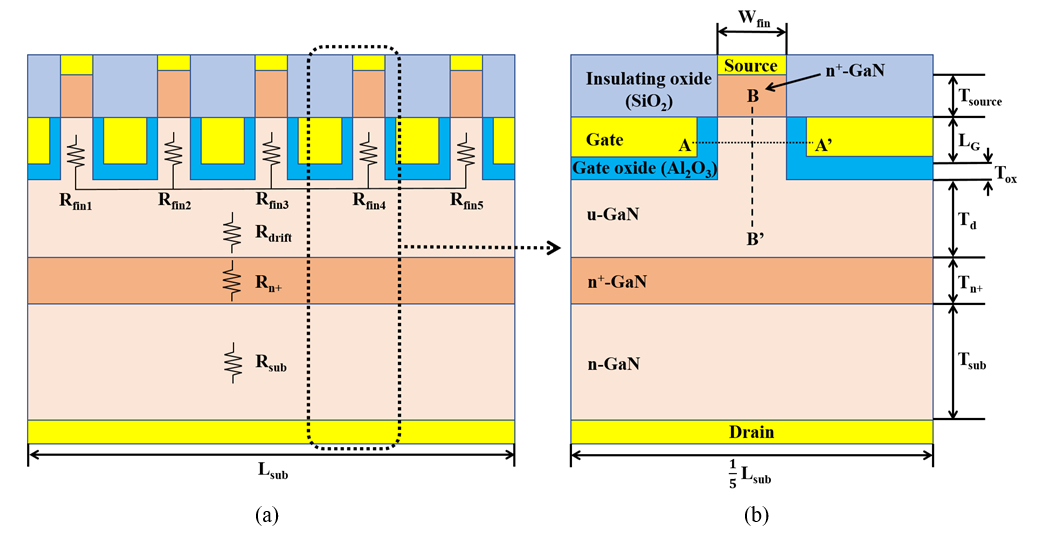##### Fig. 2. Schematics of (a) fully depleted fin channel; (b) partially depleted fin channel; (c) flat band mode; (d) accumulation mode; (e) Simulated electron concentration distribution along A-A’ cutline at different gate biases in the range of 1 to 5 V.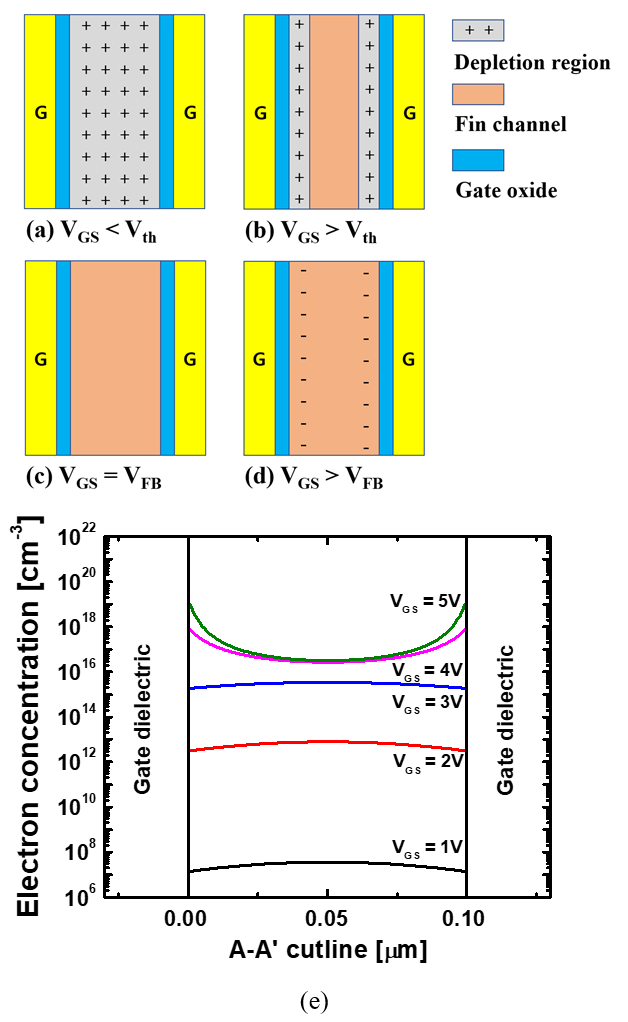##### Fig. 3. GaN/Al$_{2}$O$_{3}$ interface trap distribution.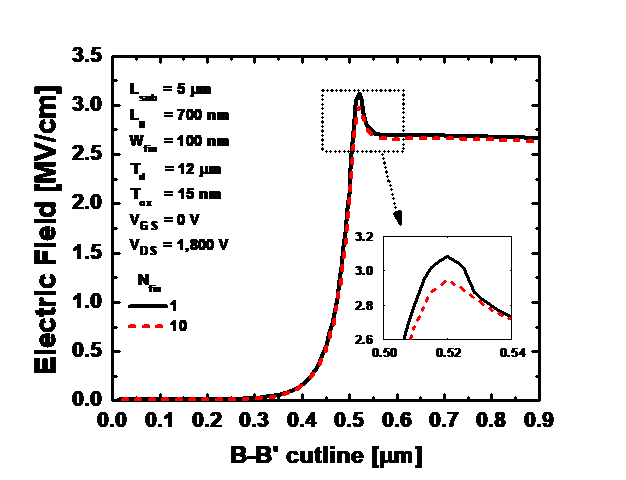## III. RESULTS AND DISCUSSION

Fig. 4(a)-(c) show the I$_{\mathrm{D}}$-V$_{\mathrm{GS}}$ transfer curve, the output current curve, and the breakdown characteristic curve of the 5-fins vertical GaN power transistor. The drain current density (I$_{\mathrm{D}}$) and the specific R$_{\mathrm{on}}$ are normalized to the total substrate area. The I$_{\mathrm{on}}$ is defined at V$_{\mathrm{GS}}$ = 6 V and V$_{\mathrm{DS}}$ = 5 V. The R$_{\mathrm{on}}$ is extracted at V$_{\mathrm{GS}}$ = 6 V and V$_{\mathrm{DS}}$ = 0.1 V. The 5-fins vertical GaN power transistor has I$_{\mathrm{on}}$ = 5.49 kA/cm$^{2}$, V$_{\mathrm{th}}$ = 3.41 V, R$_{\mathrm{on}}$ = 0.884 m${\Omega}$·cm$^{2}$, and BV = 1,999 V. Based on this result, the characteristics and advantages of the multiple fin-type vertical GaN power transistors are analyzed by comparing with those of the single-fin device.

Fig. 5(a) and (b) show I$_{\mathrm{D}}$-V$_{\mathrm{GS}}$ characteristics and transconductance according to N$_{\mathrm{fin}}$. As the N$_{\mathrm{fin}}$ increases from 1 to 10, the I$_{\mathrm{on}}$ increases from 4.87 to 5.58 kA/cm$^{2}$, and V$_{\mathrm{th}}$ decrease from 3.83 to 3.20 V. The I$_{\mathrm{on}}$ of the 10-fins device increases by 14.6% compared with that of the single-fin device, and as the N$_{\mathrm{fin}}$ increases, the increase rate of the I$_{\mathrm{on}}$ decreases significantly. Fig. 5(c) shows the BV and the R$_{\mathrm{on}}$ as a function of the N$_{\mathrm{fin}}$. As the N$_{\mathrm{fin}}$ increases from 1 to 10, the BV increases from 1,925 to 2,021 V and the R$_{\mathrm{on}}$ decreases from 1.007 to 0.867 m${\Omega}$·cm$^{2}$.

The change in the I$_{\mathrm{on}}$ and the R$_{\mathrm{on}}$ can be explained in terms of the resistance structure of the device . As shown in Fig. 1(a), the resistance (R$_{\mathrm{on,N}}$) of the N fins vertical power transistor consists of the resistances from the fins (R$_{\mathrm{finN}}$) connected in parallel with each other, the drift layer resistance (R$_{\mathrm{drift}}$), the n$^{+}$-GaN layer resistance (R$_{\mathrm{n+}}$), and the substrate resistance (R$_{\mathrm{sub}}$).

The vertical GaN Schottky barrier diode (SBD) is used to extract the resistance occupied by the fin. As presented in Fig. 6(a), the SBD has an identical u-GaN drift layer, an n$^{+}$-GaN layer, an n-GaN substrate, and a backside contact with the vertical GaN fin power transistor.

Fig. 6(b) shows the I-V curve of the vertical GaN SBD; the simulated R$_{\mathrm{SBD}}$ is 0.852 m${\Omega}$·cm$^{2}$. When simulated in the same manner, the values of R$_{\mathrm{drift}}$, R$_{\mathrm{n+}}$, and R$_{\mathrm{sub}}$ are 0.827, 0.0005, and 0.024 m${\Omega}$·cm$^{2}$, respectively. The fin resistance of the N-fins device (R$_{\mathrm{Nfin}}$) can be extracted through the difference between the on-resistance of the N-fins device (R$_{\mathrm{on,N}}$) and the resistance of the SBD (R$_{\mathrm{SBD}}$).

Determining R$_{\mathrm{Nfin}}$ by applying (3) is possible only when each fin has the same resistance. Fig. 6(c) shows the I$_{\mathrm{D}}$ of each fin of the 5-fins device along the A-A’ cutline. The current flowing through each fin is equal regardless of the location of fins. Therefore, R$_{\mathrm{fin}}$ is the same in the N-fins device. Since the number of fins does not affect the channel formation of each fin, channels formed in one fin are all the same. Therefore, the R$_{\mathrm{Nfin}}$ is constant regardless of the number of fins. The determined R$_{\mathrm{1fin}}$, R$_{\mathrm{5fin}}$, and R$_{\mathrm{10fin}}$ are 0.155, 0.160, and 0.153 m${\Omega}$·cm$^{2}$, respectively. To verify the method of determining R$_{\mathrm{fin}}$ applying (3), the simulated R$_{\mathrm{on,N}}$ and calculated R$_{\mathrm{on,N}}$ using the determined R$_{\mathrm{Nfin}}$ and R$_{\mathrm{SBD}}$ are compared, as shown in Table 2.

The change in the I$_{\mathrm{on}}$ for different values of N$_{\mathrm{fin}}$ depends on the ratio of the resistance accounted for by the fin. The relationship between the I$_{\mathrm{on}}$ and the N$_{\mathrm{fin}}$ is non-linear because the resistance of the drift layer cannot be neglected. Since the R$_{\mathrm{drift}}$ constitutes a significant portion of R$_{\mathrm{on}}$, the result shown in Fig. 5(c) appears. Fig. 6(d) shows that the I$_{\mathrm{on}}$ and the 1/R$_{\mathrm{on}}$ at different values of N$_{\mathrm{fin}}$ have a similar tendency.

The V$_{\mathrm{th}}$ shift is related to the channel formation process. Table 3 shows the I$_{\mathrm{D}}$’s for different values of N$_{\mathrm{fin}}$ at different V$_{\mathrm{GS}}$ ranging from 3.2 to 3.8 V. For the same V$_{\mathrm{GS}}$, the channel is formed equally regardless of the value of N$_{\mathrm{fin}}$ and the position of the fin. The multiple fin-type device has N$_{\mathrm{fin}}$-channels. When the depletion layer disappears and the channel begins to form, most of the resistance is applied to the fin. Therefore, before the channels are accumulated, the I$_{\mathrm{D}}$ increases as the N$_{\mathrm{fin}}$ increases, thus resulting in the V$_{\mathrm{th}}$ shift.

Even if the N$_{\mathrm{fin}}$ changes, the difference in the BV is negligible. The BV of a 10-fins device is 4.99% higher than that of the single-fin device. Fig. 7 shows the electric field along the B-B’ cutline at V$_{\mathrm{GS}}$ = 0 V and V$_{\mathrm{DS}}$ = 1,800 V. The electric field is dispersed to fins, so that the peak electric field is slightly lowered. As the electric field applied vertically is considerably affected by the vertical parameter, T$_{\mathrm{d}}$, no significant difference is observed even if N$_{\mathrm{fin}}$ is varied.

##### Table 2. Simulated R$_{\mathrm{on,N}}$ and calculated R$_{\mathrm{on,N}}$ using extracted R$_{\mathrm{Nfin}}$ and R$_{\mathrm{SBD}}$ determined at V$_{\mathrm{GS}}$ = 6 V
 Nfin Simulated Ron,N [mΩ·cm2] RSBD+R1fin/N [mΩ·cm2] RSBD+R1fin/N [mΩ·cm2] RSBD+R1fin/N [mΩ·cm2] 1 1.007 1.007 1.005 1.012 2 0.931 0.930 0.929 0.932 3 0.905 0.904 0.903 0.905 4 0.982 0.891 0.890 0.892 5 0.884 0.883 0.883 0.884 6 0.880 0.878 0.878 0.879 7 0.875 0.874 0.874 0.875 8 0.872 0.871 0.871 0.872 9 0.869 0.869 0.869 0.870 10 0.867 0.868 0.867 0.868
##### Table 3. I$_{\mathrm{D}}$ with different N$_{\mathrm{fin}}$ at different V$_{\mathrm{GS}}$ ranging from 3.2 to 3.8 V
 Nfin ID at VGS = 3.2 V [A/cm2] ID at VGS = 3.4 V [A/cm2] ID at VGS = 3.6 V [A/cm2] ID at VGS = 3.8 V [A/cm2] 1 7.89 × 101 1.84 × 102 3.52 × 102 6.30 × 102 2 1.58 × 102 3.66 × 102 7.00 × 102 1.25 × 103 3 2.36 × 102 5.49 × 102 1.05 × 103 1.85 × 103 4 3.15 × 102 7.30 × 102 1.39 × 103 2.43 × 103 5 3.92 × 102 9.10 × 102 1.72 × 103 2.98 × 103 6 4.70 × 102 1.09 × 103 2.06 × 103 3.48 × 103 7 5.46 × 102 1.26 × 103 2.38 × 103 3.92 × 103 8 6.24 × 102 1.44 × 103 2.68 × 103 4.24 × 103 9 7.00 × 102 1.61 × 103 2.98 × 103 4.50 × 103 10 7.76 × 102 1.78 × 103 3.26 × 103 4.66 × 103
##### Fig. 4. (a) I$_{\mathrm{D}}$-V$_{\mathrm{GS}}$ curve of 5-fins vertical GaN power transistor; (b) I$_{\mathrm{D}}$-V$_{\mathrm{DS}}$ curve; (c) Breakdown voltage curve at VGS = 0 V.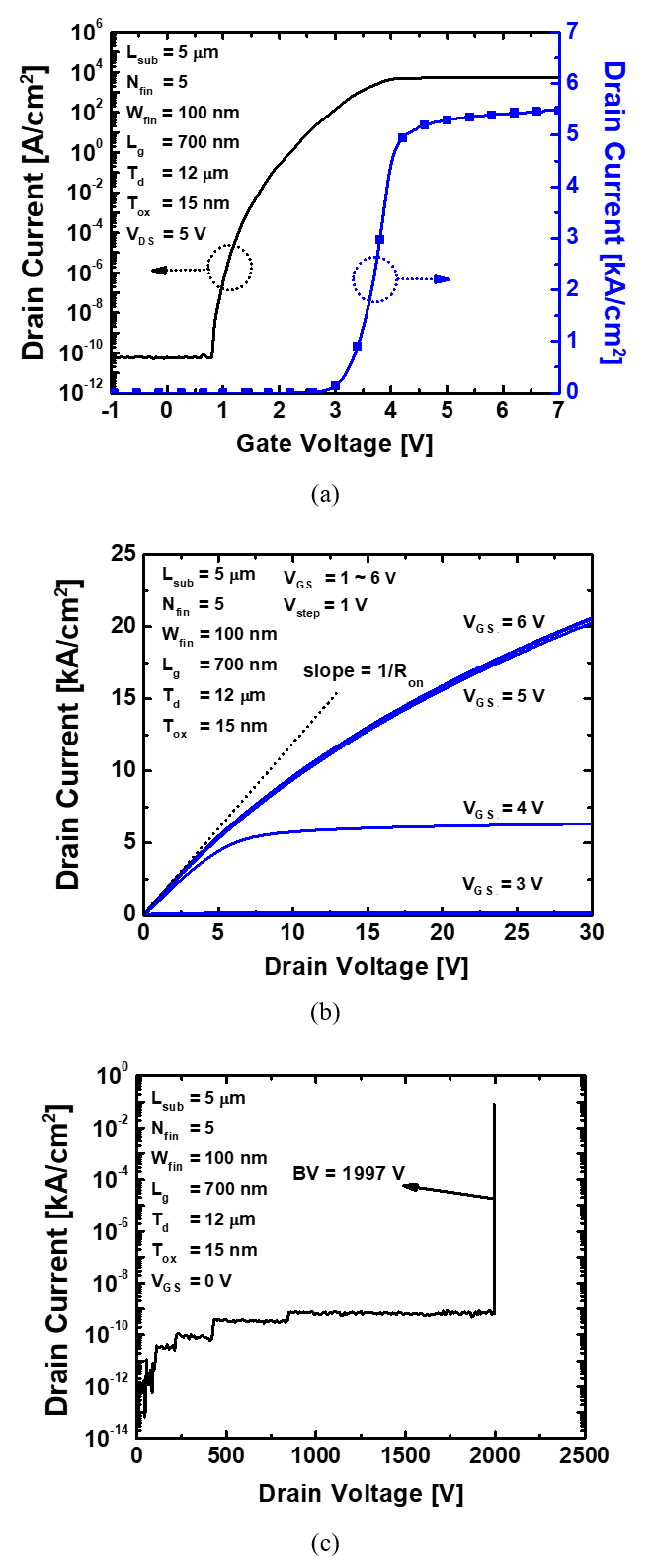##### Fig. 5. (a) I$_{\mathrm{D}}$-V$_{\mathrm{GS}}$ curve with different number of fin; (b) Transconductance; (c) Breakdown voltage (BV) and R$_{\mathrm{on}}$ as a function of the number of fin.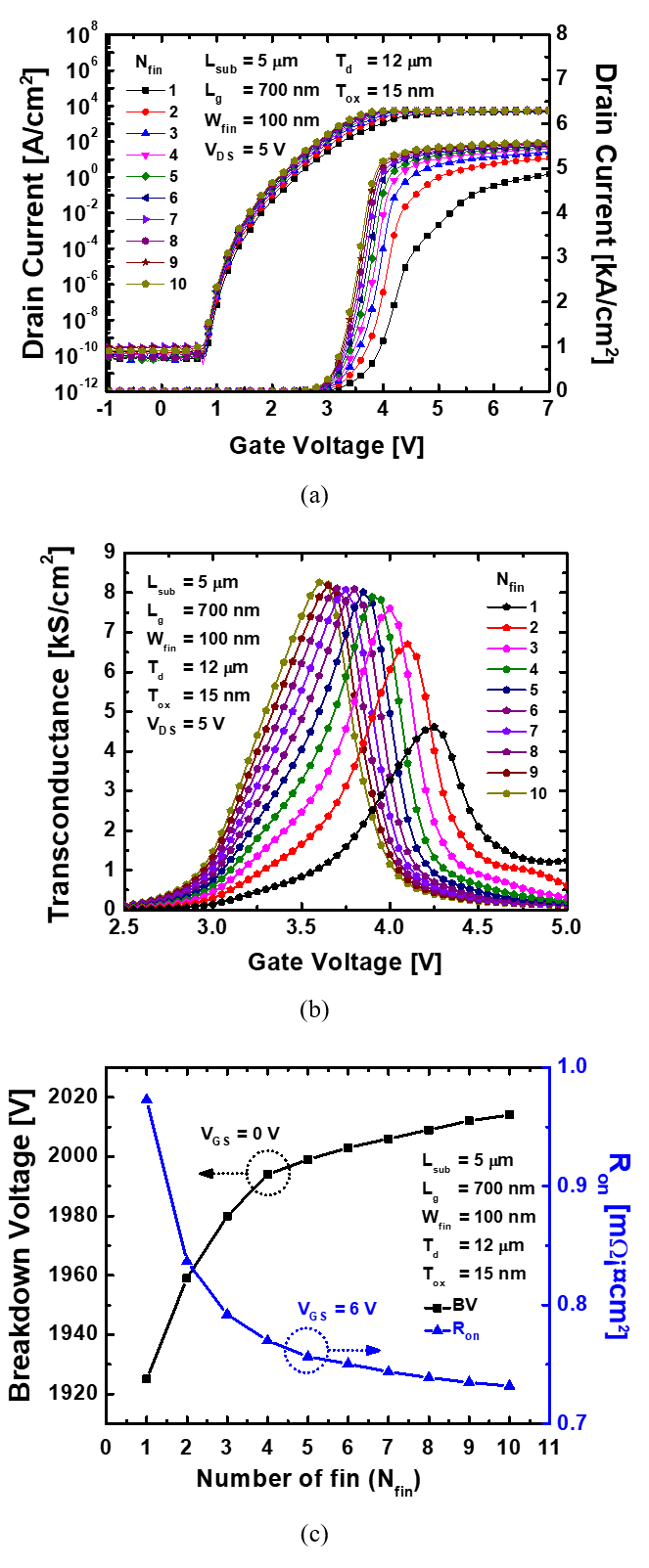##### Fig. 6. (a) Cross-sectional view of the vertical GaN Schottky barrier diode (SBD); (b) I-V curve of SBD; (c) Drain current of each fin of 5-fins device; (d) I$_{\mathrm{on}}$ and 1/R$_{\mathrm{on}}$ as a function of N$_{\mathrm{fin}}$.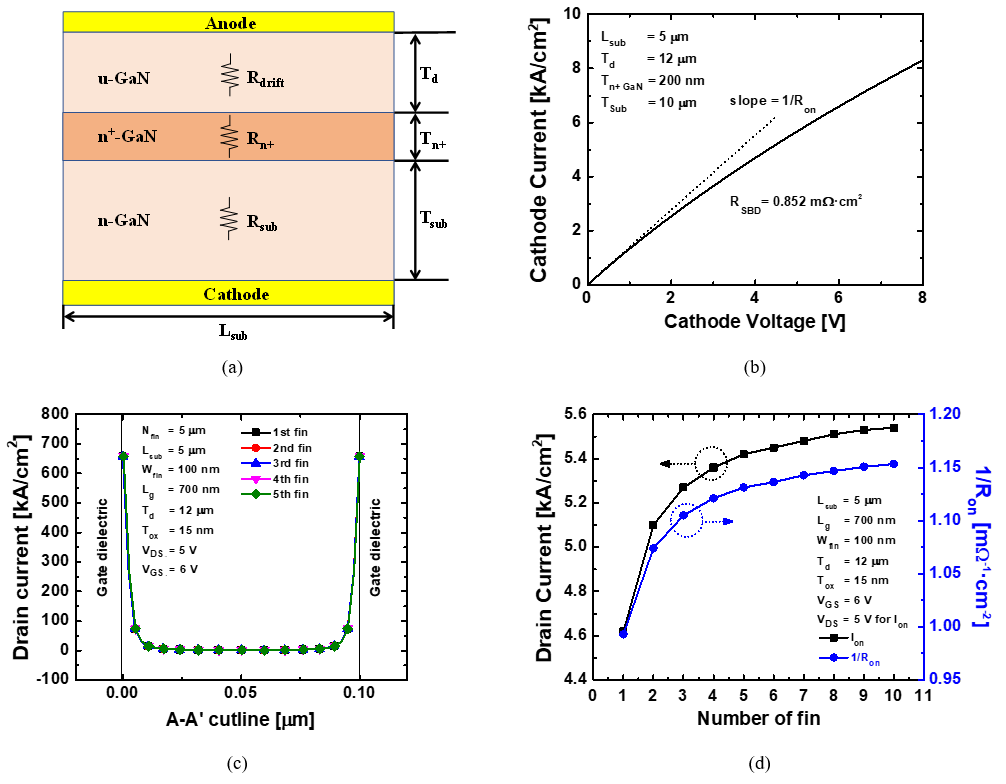##### Fig. 7. Electric field along B-B’ cutline at V$_{\mathrm{GS}}$ = 0 V and V$_{\mathrm{DS}}$ = 1,800 V.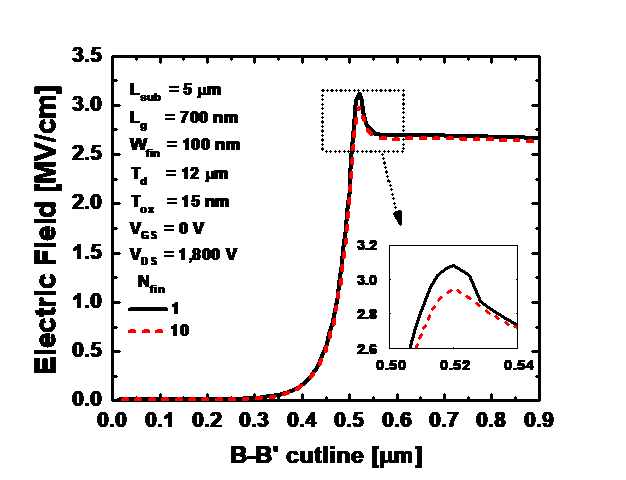## IV. CONCLUSIONS

In this study, the electrical performances of multiple fin-type vertical GaN power transistors were analyzed by conducting ATLAS TCAD simulations. In the power electronics, the large-scale power devices are required to achieve high current. Thus, the effect of increasing N$_{\mathrm{fin}}$ in the device with L$_{\mathrm{sub}}$ = 5 ${\mu}$m was investigated. As N$_{\mathrm{fin}}$ increased from 1 to 10, I\-$_{\mathrm{on}}$ increased by 14.6%, R$_{\mathrm{on}}$ decreased by 13.9%, and BV increased slightly. The multiple fin-type vertical GaN transistors showed excellent performance. The 5-fins device exhibited I$_{\mathrm{on}}$ = 5.49 kA/cm$^{2}$, V$_{\mathrm{th}}$ = 3.41 V, R$_{\mathrm{on}}$ = 0.884 m${\Omega}$·cm$^{2}$, and the BV = 1,999 V. The performance improvement decreased with an increase in N$_{\mathrm{fin}}$, and it was saturated when N$_{\mathrm{fin}}$ exceeded 5. The results of this study provide insight into the electrical characteristic of multiple fin-type vertical GaN transistors.

## ACKNOWLEDGMENTS

This work was supported by the National Research Foundation of Korea (NRF) grant funded by the Korea government (MSIT) (No. NRF-2020R1A2C1005087). This study was supported by the BK21 FOUR project funded by the Ministry of Education, Korea (4199990113966). This research was supported by Basic Science Research Program through the National Research Foundation of Korea (NRF) funded by the Ministry of Education (NRF-2021R1A6A3A13039927). This research was supported by National R&D Program through the National Research Foundation of Korea (NRF) funded by Ministry of Science and ICT (2021M3F3A2A03017764). This research was supported by National R&D Program through the National Research Foundation of Korea (NRF) funded by Ministry of Science and ICT (2022M3I7A1078936). This investigation was financially supported by Semiconductor Industry Collaborative Project between Kyungpook National University and Samsung Electronics Co. Ltd. The EDA tool was supported by the IC Design Education Center (IDEC), Korea.

## References

1
Wang, X., Huang, S., Zheng, Y., Wei, K., Chen, X., Zhang, H., & Liu, X. “Effect of GaN channel layer thickness on DC and RF performance of GaN HEMTs with composite AlGaN/GaN buffer.” IEEE Transactions on Electron Devices, vol. 61, no. 5, pp. 1341-1346, May. 2014.2
K. Hirama, M. Kasu, and Y. Taniyasu, "RF High-Power Operation of AlGaN/GaN HEMTs Epitaxially Grown on Diamond." IEEE Electron Device Letters, vol. 33, no. 4, pp. 513-515, Apr. 2012.3
Lee, J. H., Park, C., Kim, K. W., Kim, D. S, and Lee, Lee, J. H. "Performance of Fully Recessed AlGaN/GaN MOSFET Prepared on GaN Buffer Layer Grown With AlSiC Precoverage on Silicon Substrate." IEEE Electron Device Letters, vol. 34, no. 8, pp. 975-977, Aug. 2013.4
R. Chu., A. Corrion., M. Chen., R. Li., D. Wong., D. Zehnder., B. Hughes., and K. Boutros. "1200-V Normally Off GaN-on-Si Field-Effect Transistors With Low Dynamic on -Resistance." IEEE Electron Device Letters, vol. 32, no. 5, pp. 632-634, May. 2011.5
M. Ishida., T. Ueda., T. Tanaka., and D. Ueda. “GaN on Si technologies for power switching devices.” IEEE Transactions on Electron Devices, vol. 60, no. 10, pp. 3053-3059, Oct. 2013.6
M. Wang et al., "900 V/1.6 mΩ⋅cm2 Normally Off Al2O3/GaN MOSFET on Silicon Substrate." IEEE Transactions on Electron Devices, vol. 61, no. 6, pp. 2035-2040, Jun. 2014.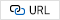7
U. K. Mishra., P. Parikh., and Yi-Feng Wu., "AlGaN/GaN HEMTs-an overview of device operation and applications." Proceedings of the IEEE, vol. 90, no. 6, pp. 1022-1031, Jun. 2002.8
J. A. del Alamo., and E. S. Lee., "Stability and Reliability of Lateral GaN Power Field-Effect Transistors." IEEE Transactions on Electron Devices, vol. 66, no. 11, pp. 4578-4590, Nov. 2019.9
G. Longobardi et al., "Impact of Donor Traps on the 2DEG and Electrical Behavior of AlGaN/GaN MISFETs." IEEE Electron Device Letters, vol. 35, no. 1, pp. 27-29, Jan. 2014.10
J. M. Tirado, J. L. Sanchez-Rojas and J. I. Izpura, "Trapping Effects in the Transient Response of AlGaN/GaN HEMT Devices." IEEE Transactions on Electron Devices, vol. 54, no. 3, pp. 410-417, Mar. 2007.11
T. Oka., and T. Nozawa., "AlGaN/GaN Recessed MIS-Gate HFET With High-Threshold-Voltage Normally-Off Operation for Power Electronics Applications." IEEE Electron Device Letters, vol. 29, no. 7, pp. 668-670, Jul. 2008.12
O. Hilt., A. Knauer., F. Brunner., E. Bahat-Treidel., and J. Würfl., "Normally-off AlGaN/GaN HFET with p-type GaN Gate and AlGaN buffer." 2010 22nd International Symposium on Power Semiconductor Devices & IC's (ISPSD), 2010, pp. 347-350.13
H. Jiang, Q. Lyu, R. Zhu, P. Xiang, K. Cheng, and K. M. Lau, "1300 V Normally-OFF p-GaN Gate HEMTs on Si With High ON-State Drain Current." in IEEE Transactions on Electron Devices, vol. 68, no. 2, pp. 653-657, Feb. 2021.14
M. Sun, Y. Zhang, X. Gao, and T. Palacios, "High-Performance GaN Vertical Fin Power Transistors on Bulk GaN Substrates." in IEEE Electron Device Letters, vol. 38, no. 4, pp. 509-512, Apr. 2017.15
M. Sun, M. Pan, X. Gao, and T. Palacios, "Vertical GaN power FET on bulk GaN substrate." 2016 74th Annual Device Research Conference (DRC), 2016, pp. 1-2.16
W. Li and S. Chowdhury, “Design and fabrication of a 1.2 kV GaN-based MOS vertical transistor for single chip normally off operation.” Phys. Status Solidi A, vol. 213, no. 10, pp. 2714-2720, Oct. 2016.17
An, H. D., Lee, S. H., Park, J. et al., “Analysis and Optimization for Characteristics of Vertical GaN Junctionless MOSFETs Depending on Specifications of GaN Substrates.” J. Electr. Eng. Technol, Jun. 2022.18
Zhang, X. Y., Yang, L. A., Yang, W. L., Li, Y., Ma, X. H., & Hao, Y. "Improved performance of Ni/GaN Schottky barrier impact ionization avalanche transit time diode with n-type GaN deep level defects." Semiconductor Science and Technology, vol. 36, no. 2, 2020.19
Mukherjee, M., Mazumder, N., Roy, S. K., & Goswami, K. "GaN IMPATT diode: a photo-sensitive high power terahertz source. " Semiconductor Science and Technology, vol. 22, no. 12, 2007.20
Jackson, C. M., Arehart, A. R., Cinkilic, E., McS-kimming, B., Speck, J. S., Ringel, S. A., “Interface trap characterization of atomic layer deposition Al2O3/GaN metal-insulator-semiconductor capaci-tors using optically and thermally based deep level spectroscopies.” Journal of Applied Physics, 133, 204505, 2013.21
M. Xiao, T. Palacios and Y. Zhang, "ON-Resistance in Vertical Power FinFETs." in IEEE Transactions on Electron Devices, vol. 66, no. 9, pp. 3903-3909, Sep. 2019.Jun Hyeok Heo received a B.Sc. degree in electronic engineering from the School of Electronics and Information Engineering, Korea University (KU) Sejong Campus, Sejong si, South Korea, in 2021, where he is currently pursuing an M.S. degree in school of Electronic and Electrical Engineering. His research interests include the design, fabrication, and characterization of vertical GaN power devices.

Sang Ho Lee received the B.Sc. degree in electronics engineering from the School of Electronics Engineering (SEE), Kyungpook National University (KNU), Daegu, South Korea, in 2019, where he is currently pursuing the Ph.D. in school of Electronic and Electrical Engineering. His research interests include the design, fabrication, and characterization of gate-all-around logic devices and capacitor-less 1T-DRAM transistors.

Jin Park received a B.Sc. degree in electronic engineering from the School of Electronics Engineering (SEE), Kyungpook National University (KNU), Daegu, South Korea, in 2020, where she is pursuing the Ph.D. in school of Electronic and Electrical Engineering. Her research interests include the design, fabrication, and characterization of gate-all-around logic devices and capacitor-less 1T-DRAM transistors.

So Ra Min received the B.Sc. degree in Electronic Engineering from the School of Electronics Engineering, Yeungnam University (YU), Gyeong-san, North Gyeongsang, South Korea, in 2020, where she is currently pursuing the M.Sc. degree in school of Electronic and Electrical Engineering. Her research interests include the design, fabrication, and characterization of GaN devices and capacitor-less 1T-DRAM transistors.

Geon Uk Kim received a B.Sc. degree in electronic engineering from the School of Electronics Engineering (SEE), Kyungpook National University (KNU), Daegu, South Korea, in 2021, where he is currently pursuing an M.S. degree in school of Electronic and Electrical Engineering. His research interests include the design, fabrication, and characterization of GaN devices and capacitor-less 1T-DRAM transistors.

Ga Eon Kang received a B.Sc. degree in electronic engineering from the School of Electronics Engi-neering (SEE), Kyungpook National University (KNU), Daegu, South Korea, in 2022, where she is currently pursuing an M.S. degree in school of Electronic and Electrical Engineering. Her research interests include the design, fabrication, and characterization of GaN devices and tunneling FETs.

Jae Won Jang received the B.S. and M.S degrees in electrical engineering from Korea University, Seoul, Korea in 2006 and 2008, respectively. In 2013, Jaewon Jang received Ph.D degrees in electrical engineering and computer sciences from University of California at Berkeley, CA, USA. From 2013 to 2014, he was a post doctorial researcher, and working for developing of high-performance metal oxide transistors by printing technology. From 2015 to 2016, he was a researcher and working for developing of high performance organic thin film transistor in Samsung Advanced Institute and Technology, Suwon, Korea. Since 2016, he has been with Kyungpook National University, Daegu, Korea, where he is currently an assistant professor with the School of Electronics Engineering.

Jin-Hyuk Bae received a B.S. degree in Electronics and Electrical Engineering from Kyungpook National University, Daegu, Korea in 2004, and M.S. and Ph.D. degrees in Electrical Engineering from the Seoul National University, Seoul, Korea in 2006 and 2010, respectively. For the period from 2010 to 2012, he worked as a post-doctoral research fellow with Ecole Nationale Superiere des Mines de Saint-Etienne, Gardanne, France. In 2012, he joined the faculty in the School of Electronics Engineering, Kyungpook National University, Korea, where he is currently an Associate Professor. His research interests include interfacial engineering and physics of organic based and metal-oxide-based electronic devices and their sensor applications.

Sin-Hyung Lee received his B.S. and Ph.D. degrees in electrical engineering from Seoul National University, Korea in 2013 and 2019, respectively. He is currently an assistant professor in the School of Electronics Engineering at Kyung-pook National University in Republic of Korea. His research covers the neuromorphic electronics, artificial synapse, memristors, and organic electronics.

In Man Kang received the B.S. degree in electronic and electrical engineering from School of Electronics and Electrical Engi-eering, Kyungpook National University (KNU), Daegu, Korea, in 2001, and the Ph.D. degree in electrical engineering from School of Electrical Engineering and Computer Science (EECS), Seoul National University (SNU), Seoul, Korea, in 2007. He worked as a teaching assistant for semiconductor process education from 2001 to 2006 at Inter-university Semiconductor Research Center (ISRC) in SNU. From 2007 to 2010, he worked as a senior engineer at Design Technology Team of Samsung Electronics Company. In 2010, he joined KNU as a full-time lecturer of the School of Electronics Engineering (SEE). Now, he is currently working as a professor. His current research interests include CMOS RF modeling, silicon nanowire devices, tunneling transistor, low-power nano CMOS, and III-V compound semiconductors. He is a member of IEEE EDS.